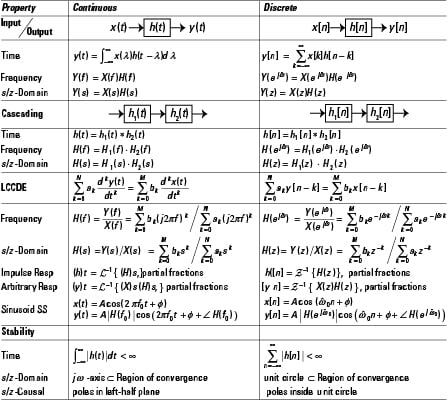##### Signals and Systems For DummiesPart of learning about signals and systems is that systems are identified according to certain properties they exhibit. Have a look at the core system classifications:

• Linearity: A linear combination of individually obtained outputs is equivalent to the output obtained by the system operating on the corresponding linear combination of inputs.

• Time-invariant: The system properties don't change with time. A present input produces the same response as it does in the future, less the time shift factor between the present and future.

• Memoryless: If the present system output depends only on the present input, the system is memoryless.

• Causal: The present system output depends at most on the present and past inputs. Future inputs can't be used to produce the present output.

• Stable: A system is bounded-input bound-output (BIBO) stable if all bounded inputs produce a bounded output.

This table presents core linear time invariant (LTI) system properties for both continuous and discrete-time systems. Time-domain, frequency-domain, and s/z-domain properties are identified for the categories basic input/output, cascading, linear constant coefficient (LCC) differential and difference equations, and BIBO stability: